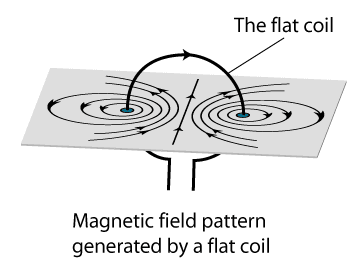## MAGNETIC FIELD DUE TO A CURRENT CARRYING CIRCULAR WIREUsing Right Hand thumb rule, the magnetic field lines at every point of the circular wire are in the form of concentric circles with wire as the center. These magnetic field lines become larger and larger as we move away from the wire. Just at the center of the coil, magnetic field lines are almost straight. By applying Right hand rule, it is easy to check that every section of the wire contributes to magnetic field lines in the same direction within the loop.

## Factors on which the magnetic field at the centre of the circular loop depends upon

1.   Directly proportional to the current flowing in the loop.
2.   Inversely proportional to the radius of the circular wire.
3.   Directly proportional to the number of turns of the circular loop. This is because the current in each circular turn has the same direction, and the field due to each turn then just adds up.

4.   Solenoid